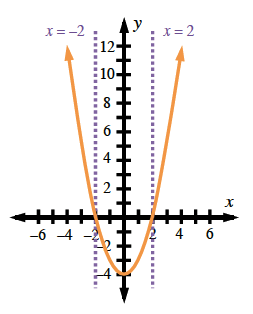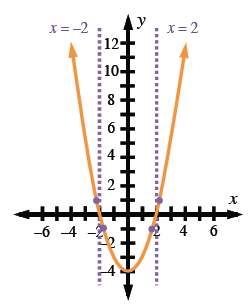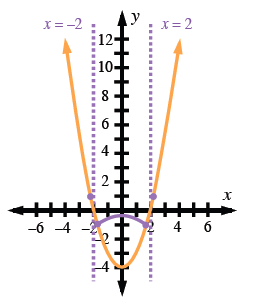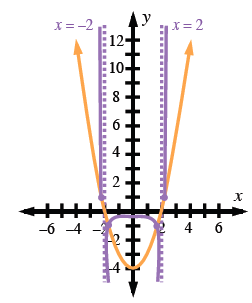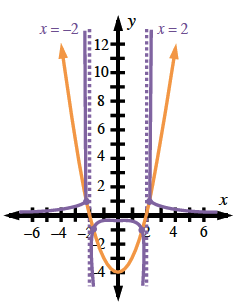### Home > PC > Chapter 5 > Lesson 5.2.3 > Problem5-74

5-74.

Use the graph of $y = x^{2} − 4$ to sketch the graph of $f ( x ) = \frac { 1 } { x ^ { 2 } - 4 }$ without using a graphing calculator. State the equations of the asymptotes in the graph of $f\left(x\right)$Graph the $y =$ function and identify the zeros ($x$-intercepts).
For the reciprocal function, $\frac{1}{0}$ is undefined, so these are the locations of the vertical asymptotes.
Sketch the vertical asymptotes.

Upward parabola, vertex at (0, comma negative 4), x intercepts at negative 2 & 2, dashed vertical segments at x = negative 2 and x = 2.

Mark the places where $y = 1$ or $−1$. These points do not change because $\frac{1}{1} = 1$ and $\frac{1}{−1} = −1$.

Added approximate highlighted points on parabola, (negative 2.2, comma 1), (2.2, comma 1), (negative 1.7, comma negative 1), & (1.7, comma negative 1).

The reciprocal at the point $\left(0, −4\right)$ is $\left(0, −\frac{1}{4}\right)$. Mark it.
Draw a smooth curve from it to where $y = −1$ is marked and to where $y = 1$ is marked.

Added, downward curve between highlighted points below x axis, going through the point (0, comma 1 fourth).

When the $y$-values of the original function are small, $\frac{1}{\left(± \text{ small number}\right)}$ is a $±$ large number. Show this on your graph.

Added, 4 almost vertical curves, from each of the highlighted points above x axis, to infinity, and from each of the highlighted points below the x axis, to negative infinity.

When the $y$-values of the original function are large, $\frac{1}{\text{large number}}$ is a small number. Show this on your graph.

Added, upward curves from approximate point, (negative 2.2, comma 1), going left above x axis, from approximate point (2.2, comma 1), going right above x axis.